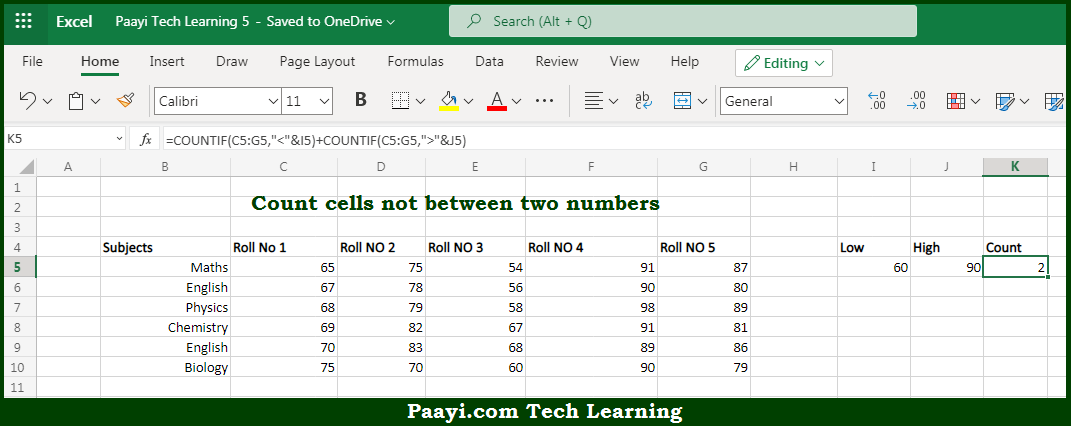# Learn How to Count Cells Not Between Two Numbers In Microsoft Excel

Written by | 0 Comments | 598 Views

In this article, you will learn how to use the Microsoft Excel COUNT formula and its purpose in Microsoft Excel. You will also get to know how to count cells, not between two numbers, and see the generic formula.

Count Cells Not Between Two Numbers Using Microsoft Excel Count Formula

The main purpose of the Microsoft Excel COUNT formula is to count cell values that are not between two numbers. Here we will learn how to count cells between two numbers.  That implies, with the help of the COUNT formula you can able to count cell values that are not between two numbers, you can use the COUNTIF function. At each new row, this formula returns a count of values not between the low and high values. So, with the help of the COUNT formula, you can able to count cell values that are not between two numbers.

General Syntax of the COUNT Cells Count Cells Less than Others

=COUNTIF(range,"<"&low)+COUNTIF(range,">"&high)

The Explanation for the Count Cells Less than OthersSo we know that Microsoft Excel COUNT formula you can able to count cell values that are not between two numbers. Here we will learn how to count cells between two numbers. The main purpose of the example provided here is to count the number of values recorded over five days. These five days do not fall between two numbers, a low value, and a high value. It can be understood as, to count values that are "out of range".

It should be noted that you can also use the SUMPRODUCT function for this. So, with the help of the COUNT formula, you can able to count the number of cells that contain values less than a particular number.